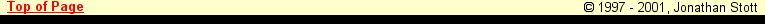Sponsored by the
Institute of PhysicsConstantsEquationsPeriodic TableConversionsJava StructurerSI UnitsSymbols
Greek Alphabet
Mathematical Symbols
Electronic Symbols
Dalton's Table of Elements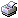Printer Version
Science
Symbols - Mathematical Symbols

 + plus/add - minus/subtract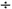divide / divide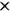multiply/times . multiply/times, scalar product of vectorsis equal tois identically equal to, defined as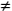does not equalis approximately equal tois greater than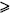is greater than or equal to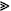is much greater than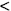is less thanis less than or equal tois much less thanplus or minus, error margin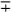minus or plus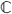complex setinteger setnatural setrational setreal setis to, ratio, such that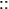asthereforebecause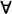for all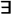there exists { } set < > mean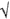(square) root ofdenotes an operationangleparallelcongruentperpendicular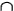intersectionunionsubset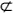is not a subset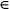belongs todoes not belong toempty set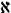cardinality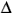finite difference or incrementcolonsemicolon % per cent ' first derivative, feet, arcminutes " second derivative, inches, arcseconds ° degreesdifferenceellipsis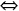is equivalent toimpliesfactorialinfinityintegralmaps into, approaches the limitthe sum of the terms indicated (sigma)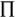the product of the terms indicated (pi)is proportional to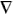vector differential

[ H O M E ] [ S C I E N C E ] [ M A T H S ] [ A S T R O N O M Y ]
Download | Shop | Contact | About

Page compiled: Wed Sep 05 18:37:57 GMT+01:00 2001